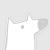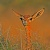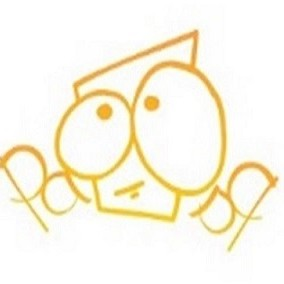IEEE\RSJ International Conference on Intelligent Robots and Systems（IROS 2019）包括全体会议和主题演讲、技术会议、研讨会和教程、论坛、先驱演讲和展览，以丰富与会人员之间富有成果的讨论。它是机器人与智能系统领域的旗舰国际会议，由IEEE、IEEE机器人与自动化协会（RAS）、IEEE工业电子协会（IES）、日本机器人协会（RSJ）、仪器与控制工程师协会（SICE）和新技术基金会（NTF）共同赞助。IEEE是一个非盈利的技术专业协会，在160个国家拥有40多万会员。它是从计算机工程、生物医学技术和电信到电力、航空航天和消费电子等技术领域的领先权威。官网链接：https://www.iros2019.org/0+0+0+0+0+0+0+0+0+0+0+0+0+0+0+0+0+0+0+0+0+0+0+0+0+1+0+0+0+0+0+0+0+0+0+0+0+1+0+0+0+0+0+0+0+
Top